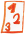Custom math worksheets at your fingertips# Details for problem "Percent base value calculation from base and rate"

Quickname: 6679

## Summary

Percent rate and percentage are given, calculate the rate and fill in the empty box.

## Example## Description

A series of percentage calculation terms is shown. The base value has been replaced by a blank space.

The base and percentage values are given. The base value is within a choosable number range. It may be a whole number, or a decimal fraction. For the decimal fraction case, the number of decimal places is selectable.

From the values given, the base value has the be calculated and put in the empty box.

The rate is in the 0 to 100 percent range. A raster of 0.1%, 1%, 5% and 10% can be selected for the rate.

The number of problems is selectable.

Download free worksheets for this math problem here. There is a second sheet with the solutions. Just click on the respective link.

•Worksheet 1Solution sheet
•Worksheet 2Solution sheet
•Worksheet 3Solution sheet

If you can not see the solution sheets for download, they may be filtered out by an ad blocker that you may have installed. If this is the case, please allow ads for this page and reload the page. The solution sheets will then reappear.

• Do the sample worksheets do not really fit?
• Do you need more math worksheets, with a different level of difficulty?
• Would you like to combine different problems on a worksheet and adjust them to your needs?
• As a teacher, you can put together your own worksheets using the automatically generated math problems provided.
With a free initial credit, you can start creating your own math worksheets in a few minutes.

It does not cost anything to try! Register here, to create custom worksheets now!

## Customization options for this problem

Parameter
Possible values
Number of problems
1, 2, 3, 4, 5, 6, 7, 8, 9, 10
Number range
20, 50, 100, 200, 500, 1000, 10000, 100000, 1000000, 10000000
Decimal places
0, 1, 2, 3
Rate raster
0.1%, 1%, 5%, 10%

## Similar problems

Remark
Description
mixed questions asking for base, rate or percentage
In a percentage term, one of the base, percentage, or percent rate has to be filled in.
similar, but calculate the rate
Base value and percentage are given, calculate the rate and fill in the empty box.
similar, but calculate the percentage
Calculate percentage from base value and rate.

Deutsche Version dieser Aufgabe
These informational pages with samples describe math problems that can be combined on custom math worksheets with solutions for home and school use.